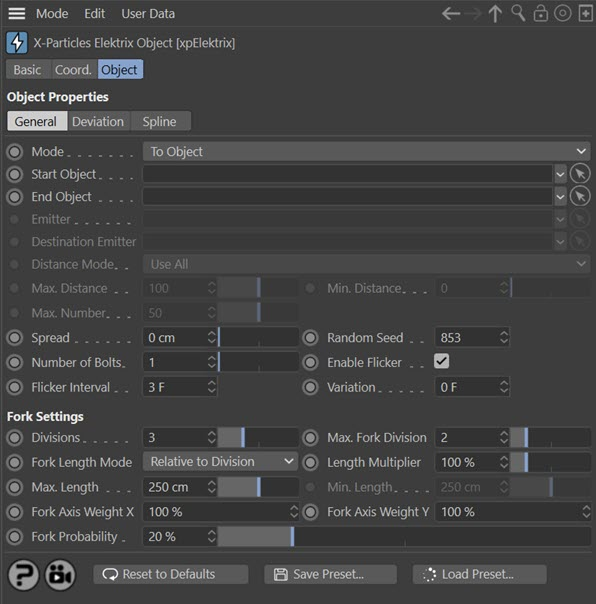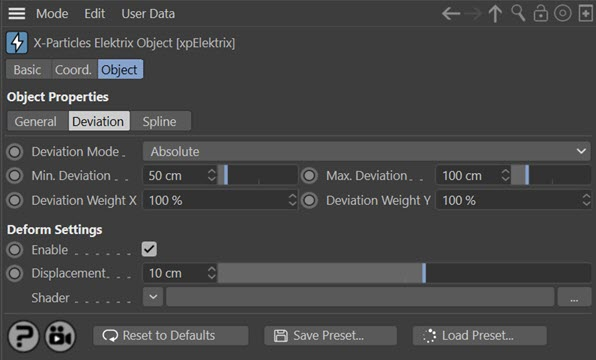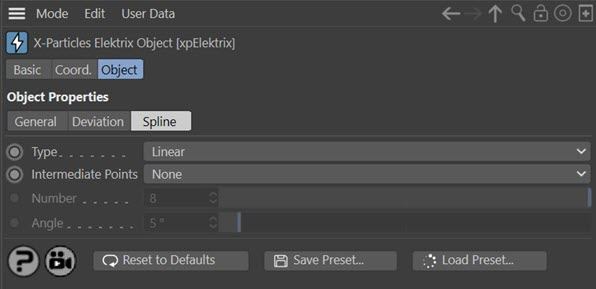# Elektrix Object

This is a special object in X-Particles which creates 'lightning bolt' style effects. Unlike other X-Particles objects, it can be used with or without a particle emitter.

## Interface

The object's interface includes these sections:

For the buttons at the bottom of the interface, please see the 'Common interface elements' page.

## General quicktab### Mode

The Elektrix object has four modes of operation:

#### To Object

With this mode you do not need a particle emitter. Instead the bolt is drawn between two objects. These can be any object, including lights or null objects. The bolt is drawn from the axis position of the 'Start Object' to the axis position of the 'End Object'.

#### Spherical

Again, this does not require an emitter. The bolt is drawn from the 'Start Object' axis position to a randomly-chosen position on an invisible sphere surrounding the 'Start Object'. The length of the bolt is governed by the 'Bolt Length' and 'Variation' settings.

#### To Particles

In this mode a bolt is drawn from the the 'Start Object' axis position to each particle emitted from an emitter.

#### Particle To Particle

Here, bolts are drawn from particles in one emitter to the corresponding particle in the 'Destination Emitter'. By 'corresponding' we mean that bolts are drawn between particle 1 in the first emitter to particle 1 in the second, and so on. It is your responsibility to make sure that the destination emitter has enough particles to match those emitted from the first emitter.

### Start Object

The object the bolt originates from in all but 'Particle To Particle' mode. Drag the object to be used into this field.

### End Object

The object the bolt ends at in 'To Object' mode. Drag the object to be used into this field.

### Emitter

The source emitter used in 'To Particle' and 'Particle To Particle' modes. Drag an X-Particles emitter into this field.

### Destination Emitter

The emitter providing the 'destination' particles in 'Particle To Particle' mode. Drag an X-Particles emitter into this field. It cannot be the same as the first emitter; the object will not generate bolts if it is.

### Distance Mode

This drop-down menu is only used when 'Mode' is set to 'To Particles' or 'Particle to Particle'. It has the following options:

#### Use All

Bolts will be drawn to all particles. This is the same behaviour as in previous versions of Elektrix.

#### Nearest Only

A bolt will only be drawn to the nearest particle.

#### All Within Distance

Bolts will be drawn to all particles whose distance is between the values in 'Max. Distance' and 'Min. Distance'.

#### Max. Number Within Distance

This is the same as 'All Within Distance' but the number of bolts is limited to the value in 'Max. Number'.

### Max. Distance

This is the farthest away a particle can be from an object or a particle for a bolt to form when 'Distance Mode' is set to 'All Within Distance' or 'Max. Number Within Distance'.

### Min. Distance

This is the closest a particle can be to an object or a particle for a bolt to form when 'Distance Mode' is set to 'All Within Distance' or 'Max. Number Within Distance'.

### Max. Number

When 'Mode' is set to 'Max. Number Within Distance' this is the maximum number of bolts which can form.

This is only used in 'To Object' mode. If it is set to zero, the end position of all the bolts will be at the axis position of the 'End Object'. Increasing this value will randomly spread the end position around the axis position of the 'End Object', within this distance value.

### Random Seed

This is the seed used when generating the bolts. If you don't like the result, try changing this value.

### Number of Bolts

Only used in 'To Object' and 'Spherical' modes, as in the other modes the number of bolts is determined by the number of particles. It controls the number of bolts which are generated.

### Enable Flicker

If checked, each bolt will randomly flick to another shape at intervals determined by the 'Flicker Interval' setting. If you don't want this to happen, uncheck this switch.

### Flicker Interval and Variation

The time between shape changes in each bolt. Variation can be added with the 'Variation' setting to prevent too regular a result. Only available if 'Enable Flicker' is checked.

### Divisions

This controls the subdivision of the bolt. A value of zero simply generates a straight line between the two points. When set to 1, the bolt will be divided in two somewhere along its length, causing a bend in the bolt (see 'Deviation Mode' below). Increasing the count to 2 will divide each half of the bolt in two again. Each time the number of divisions increases, the number of segments in the bolt is doubled. The bolt will remain the same general overall shape, but the detail will increase significantly. A value of 4 - 6 is fine for most purposes.

Note that each time a division occurs a new point is generated in the spline which the object creates. At high division levels and with many bolts this can result in a lot of points!

### Max. Fork Division

As well as dividing the bolt segments in two at each division, the object can also produce forks or branches from the point of division. If you have 6 divisions you may end up with a lot of little forks, so this setting halts forking at the level of division in this setting. A value of 3 is usually sufficient. A value of zero will prevent all forking.

Note that this value can never exceed the value in 'Divisions'.

### Fork Length Mode

Note that this setting refers to the length of any forks in the bolt, not the length of the bolt itself.

How long should a fork be? The default is that when a fork is generated, it is the length of the distance between the point it is generated from and the next point in the spline. As the bolt proceeds through the number of iterations (divisions) in the object, the distance between spline points gets shorter and shorter. The result is a few long forks and a larger number of small forks.

The alternative mode is that the fork has an absolute length in scene units. In this mode all the forks have a variable length between a maximum and minimum value.

This drop-down enables you to choose the mode to use.

#### Relative to Division

This is the default mode where the fork length depends on the division level at which it is generated.

#### Absolute

The alternative mode, in which the length is an absolute value in scene units.

### Length Multiplier

Regardless of length mode, this value is used to multiply the fork length. All forks will then show a uniform increase or decrease in length. Note that, in 'Relative to Division' mode the length will still be clamped at a maximum value as shown in the 'Max. Length' setting.

### Max. Length

In 'Relative to Division' mode, this setting ensures that the fork length never exceeds this value. If the fork length is lower than this value, it is unaffected.

In 'Absolute' mode, this is the upper limit of the length of the fork and is used in conjunction with the 'Min. Length' setting to determine the actual length.

### Min. Length

Used only in 'Absolute' mode, this is the lower limit of the length of the fork. It is used together with the 'Max. Length' setting.

### Fork Axis Weight X and Fork Axis Weight Y

When the fork is generated it deviates from its parent along the X and Y axes local to the object. If both values are set to 100% the fork may deviate away at any angle. If you set the Y value (for example) to 0%, the fork will only deviate along the local X axis.

For the most part you can leave these values at the default of 100% for a truly random deviation.

### Fork Probability

This the probability that a fork will occur at a division point. You can alter the value here to increase or reduce the chance of forking. Generally, smaller numbers produce better results.

### Bolt Length and Variation

These two settings are only available in 'Spherical' mode and control the length of the bolt. Variation can be added to the length with the 'Variation' setting.

## Deviation quicktab### Deviation Mode

This drop-down controls the amount of deviation of each point in the bolt - that is, it controls the amount of 'bending' at each division point. It has two settings:

#### Absolute

The amount of deviation is an absolute value in scene units.

#### Proportionate to Length

In this mode the amount of deviation is a proportion of the bolt length. The reason for this is that if the bolt length is small, the amount of deviation in 'Absolute' mode may be very disproportionate to the bolt length. This mode will adjust the deviation according to the length of the bolt, ensuring a more consistent appearance if the bolt length changes, for example in either of the particle modes.

### Min. Deviation, Max. Deviation

The amount of deviation or bending at each division point. These are either in scene units ('Absolute' mode) or as a percentage of the bolt length ('Proportionate to Length' mode).

### Deviation Weight X and Deviation Weight Y

When the fork deviates from its parent it does so along the X and Y axes local to the object. If both of these values are set to 100% the fork may deviate away at any angle. If you set the Y value (for example) to 0%, the fork will only deviate along the local X axis.

For the most part you can leave these values at the default of 100% for a truly random deviation.

### Deform Settings

These settings allow you to add additional deformation to the bolt with a shader. Best results are obtained with an animated Noise shader but any shader can be used.

#### Enable

If checked, the shader will deform the bolt.

#### Displacement

This is the 'strength' of the deformation. Larger values will result in larger deformation. If it is too large you may see artefacts such as forks becoming detached from their parent branch.